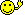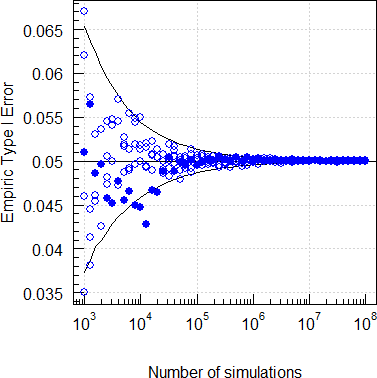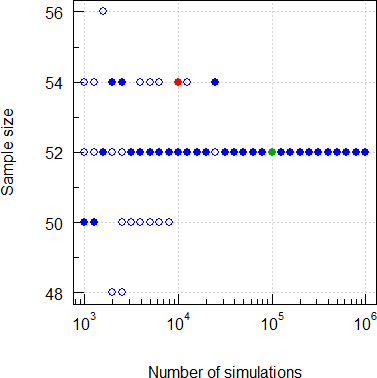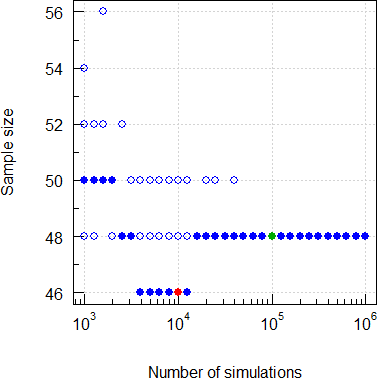## Simulations 101 [Power / Sample Size]

Dear all,

off-list I was asked about some discrepancies between sample sizes estimated by PowerTOST’s functions sampleN.scABEL(), sampleN.RSABE() and the tables of the Two Lászlós1 (Charlie).

Excursion: An introduction toMonte Carlo Simulations.

With a reasonably large number of simulations the empiric value will converge to the true value. How fast a sufficiently accurate result is obtained, depends on the problem. Hence, it is a good idea to assess that before you set the number of simulations. See IV and V below why we opted for 100,000 as the default in PowerTOST.

1. Rolling two dice
Here it’s easy to get the average sum of pips. If you remember probability and combinatorics, you will get the answer 7. If you are a gambler, you simply know it. If you have two handy, roll them. Of course, you will get anything between the possible extremes (2 and 12) in a single roll. But you will need many rolls to come close to the average 7. Since this is a boring excercise (unless you find an idiot betting against 7), letdo the job:2. Estimating π
We generate a set of uniformly distributed coordinates {x|y} in the range {–1, +1}. According to Mr Pythagoras all x2 + y2 ≤ 1 are at or within theunit circle and coded in blue. Since $$A = \frac{\pi \, d^2}{4}$$ and $$d = 1$$, we get an estimate of π by 4 times the number of coded points (the “area”) divided by the number of simulations. Here for 50,000:This was a lucky punch since the convergence is lousy. To get a stable estimate with just four significant digits, we need at least 100 million (‼) simulations. Not very efficient.

3. Type I Error of TOST
We know that α (probability of the Type I Error) never exceeds the nominal level of the test (generally 0.05). In e.g., a 2×2×2 crossover with CV 20% and 20 subjects it is 0.049999895… We can simulate studies with a GMR at one of the borders of the acceptance range and assess them for ABE as well. We expect ≤5% to pass. The number of passing studies divided by the number of simulations gives the empiric Type I Error. I used the function power.TOST.sim().The filled circles are runs with a fixed seed of 123456 and open circles ones with random seeds.
We see that convergence of this problem is bad. Therefore, e.g., in Two-Stage Designs 106 simulations are common.

4. Sample size for ABEL (EMA)
Table A21 gives for a 4-period full replicate study (EMA method), GMR 0.85, CV 50%, and ≥80% power a sample size of 54, whilst sampleN.scABEL() gives only 52.Five runs. As before filled circles are with a fixed seed (default) and open circles with random seeds.
With sampleN.scABEL() and only 10,000 simulations I got* five times 52 and once 54 (like The Lászlós). If you have more runs, you will get in some of them more extreme ones (in 1,000 runs of 10,000 I got x̃ 52 and a range of 48–56). However, this range shows that the result is not stable. Hence, we need more simulations to end up with 52 as a stable result. Although you are free to opt for more than 100,000 it’s a waste of time.

5. Sample size for RSABE (FDA)
Table A41 gives for a 4-period full replicate study (FDA method), GMR 0.90, CV 70%, and ≥90% power a sample size of only 46, whilst sampleN.RSABE() gives 48.Filled circles are with a fixed seed (default) and open circles with random seeds.
With sampleN.RSABE() and 10,000 simulations I got* once 46 (like The Lászlós), once 48, and thrice 50. In 1,000 runs of 10,000 I got x̃ 48 and a range of 44–52. With more simulations we end up with 48 as a stable result.

László Tóthfalusi coded in MatLab. Though – like in– theMersenne Twister is the standard (pseudo-random) number generator, its implementation might differ. Even with the same seed and the same number of simulations different results may be obtained.
For a comparison with the tables, execute the scripts test_ABEL.R and test_RSABE.R in the folder /library/PowerTOST/tests. Patience, please; esp. the first one takes ages to complete. If you want to get a faster result, change in test_ABEL.R lines 71 and 80 from sdsims = TRUE to sdsims = FALSE.
Another discrepancy arises from the fact that all sample size functions of PowerTOST return balanced sequences, whereas the Two Lászlós reported the smallest sample size which gives at least the desired power. Therefore, in Tables A1/A3 you find some even numbers (for three sequences) and in Tables A2/A4 some odd numbers (for two sequences), whereas sampleN.RSABE() and sampleN.scABEL() will round up to the next multiple of three and two, respectively.
The Two Lászlós simulated subjects, whereas in sampleN.RSABE()/sampleN.scABEL() the ‘key’ statistics2 are used (GMR lognormal-distributed and s2 χ2-distributed). The latter approach is easily 100 times faster than the former.
• For ABEL subject simulations are provided by the function sampleN.scABEL.sdsims(). Only if you suspect heterogenicity (i.e., give CV as a vector with two elements, where the first one is CVwT and the second CVwR), you plan a partial replicate design, and CVwT > CVwR, this is the method of choice. If CVwT ≤ CVwR and for full replicate designs we recommend sampleN.scABEL() for speed reasons. See also the vignette.
• Sorry, subject simulations are not  implemented  possible for the FDA’s RSABE. Since the FDA’s mixed-effects model for ABE (applicable if swR < 0.294) cannot be set up in, intra-subject contrasts are used. However, it is a reasonably close approximation. For a comparison with SAS see page 16 of the file Implementation_scaledABE_sims.pdf in the folder /library/PowerTOST/doc.
Furthermore, likely they used the rounded regulatory constant $$\small{0.893}$$ and not the exact $$\small{\log_{e}(1.25)/0.25=0.8925742\ldots}$$ like sampleN.RSABE(). You can try
reg         <- reg_const("FDA") reg$r_const <- 0.893 reg$name    <- "The Laszlos\u2019 likely"
and call sampleN.RSABE(..., regulator = reg). In re-calculating the entire tables with 100,000 sim’s I found five of the 352 scenarios where the sample size would be by one sequence smaller. However, I would not go that way because if you will evaluate the study with the SAS-code of the progesterone guidance or the template for Phoenix/WinNonlin, the exact regulatory constant will be used.
Another story is at which CV (on the average) we will switch in the simulations. You can try the following code to compare sample sizes for ABE and RSABE:
library(PowerTOST) fun <- function(x) {   n.1 <- sampleN.TOST(CV = x, theta0 = theta0, targetpower = target,                       design = design, details = FALSE,                       print = FALSE)[["Sample size"]]   n.2 <- sampleN.RSABE(CV = x, theta0 = theta0, targetpower = target,                        design = design, details = FALSE,                        print = FALSE)[["Sample size"]]   return(n.1- n.2) } theta0 <- 0.9 target <- 0.8 design <- "2x2x4" cat("Equal sample sizes for ABE and RSABE at CV \u2264",     signif(uniroot(fun, interval=c(0.2, 0.35),     extendInt="yes")\$root, 3), "\n")
Given that, it hardly matters for true HVD(P)s. Note that the tables of the Two Lászlós don’t reach below 30% and they stated in the discussion:
• In view of the consequences of the mixed approach, it could be judicious to consider larger numbers of subjects at variations fairly close to 30%.

If you want to reproduce simulations, keep the default argument sampleN.foo(..., setseed = TRUE). Recommended, unless you want to assess the performance and convergence. However, results with 100,000 simulations are extremely stable. For the ABEL example I got in 1,000 runs with random seeds 995 times 52 and just five times 54.

1. Tóthfalusi L, Endrényi L. Sample Sizes for Designing Bioequivalence Studies for Highly Variable Drugs. J Pharm Pharmaceut Sci. 2011;15(1):73–84.Open access.
2. Zheng C, Wang J, Zhao L. Testing bioequivalence for multiple formulations with power and sample size calculations. Pharmaceut. Stat. 2012;11(4):334–341. doi:10.1002/pst.1522.

• If you perform five runs with 10,000 simulations, you might get different numbers. That’s the effect of random seeds and a low number of simulations.

Dif-tor heh smusma 🖖
Helmut SchützThe quality of responses received is directly proportional to the quality of the question asked. 🚮
Science QuotesIng. Helmut Schütz